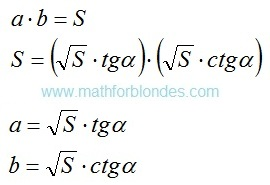## 9/03/2016

### Decomposition on factors

Subject of occupations:
TRIGONOMETRIC FUNCTIONS IN A RECTANGLE
Subject of the previous lesson
Zero and infinity

Lesson 12

Decomposition on factors

Mathematical operation, opposite on sense to multiplication, decomposition on factors is. It is carried out with application of the infinite trigonometric functions.Decomposition on factors
The most prime example of decomposition on factors at an angle in 45 degrees is root squaring. As both factors in this case are identical, as result of decomposition it is accepted to write down only one of factors.

Decomposition on factors can be applied when only the result of multiplication is known and any of factors is not known. Units of measure as a result decomposition on factors should be selected intuitively so that as a result of their multiplication the tentative unit of measure turned out. The quantity of spacelike dimensions in units of measure of factors at decomposition can be a miscellaneous. For example, three-dimensional volume can be spread out to one-dimensional factors by means of two operations of decomposition, one of options looks so:Decomposition of volume

In this example corners α and β are not bound among themselves. If to display volume in a cube (a=b=c), then α≈35° is a angle between the diagonal of a cube and diagonal of the basis, β=45 ° is a angle between the diagonal of the basis and its party.

At the following lesson we will consider
Division